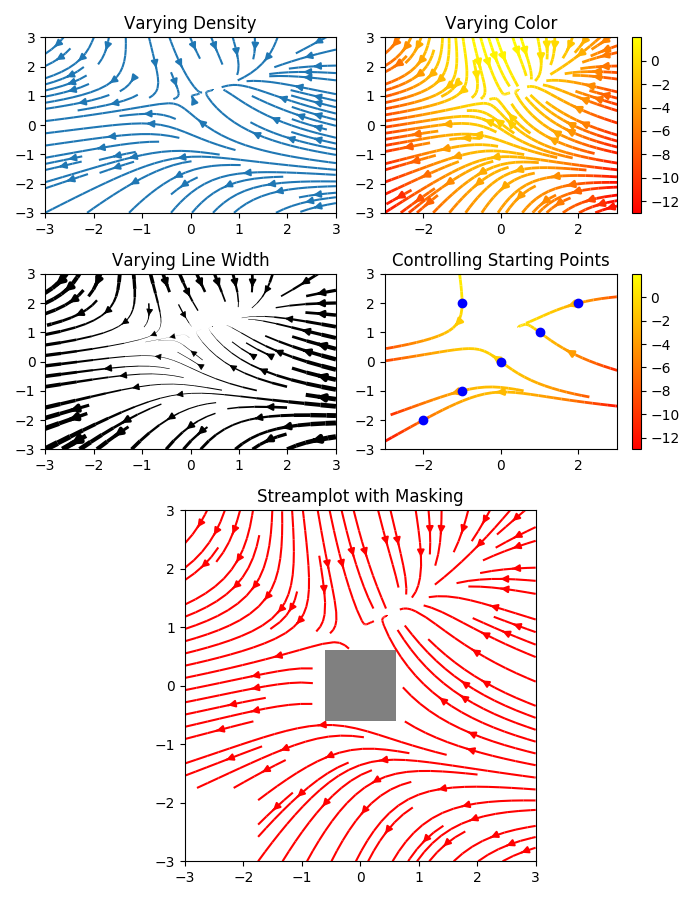# Streamplot¶

A stream plot, or streamline plot, is used to display 2D vector fields. This example shows a few features of the streamplot function:

• Varying the color along a streamline.
• Varying the density of streamlines.
• Varying the line width along a streamline.
• Controlling the starting points of streamlines.
• Streamlines skipping masked regions and NaN values.```import numpy as np
import matplotlib.pyplot as plt
import matplotlib.gridspec as gridspec

w = 3
Y, X = np.mgrid[-w:w:100j, -w:w:100j]
U = -1 - X**2 + Y
V = 1 + X - Y**2
speed = np.sqrt(U*U + V*V)

fig = plt.figure(figsize=(7, 9))
gs = gridspec.GridSpec(nrows=3, ncols=2, height_ratios=[1, 1, 2])

#  Varying density along a streamline
ax0.streamplot(X, Y, U, V, density=[0.5, 1])
ax0.set_title('Varying Density')

# Varying color along a streamline
strm = ax1.streamplot(X, Y, U, V, color=U, linewidth=2, cmap='autumn')
fig.colorbar(strm.lines)
ax1.set_title('Varying Color')

#  Varying line width along a streamline
lw = 5*speed / speed.max()
ax2.streamplot(X, Y, U, V, density=0.6, color='k', linewidth=lw)
ax2.set_title('Varying Line Width')

# Controlling the starting points of the streamlines
seed_points = np.array([[-2, -1, 0, 1, 2, -1], [-2, -1,  0, 1, 2, 2]])

strm = ax3.streamplot(X, Y, U, V, color=U, linewidth=2,
cmap='autumn', start_points=seed_points.T)
fig.colorbar(strm.lines)
ax3.set_title('Controlling Starting Points')

# Displaying the starting points with blue symbols.
ax3.plot(seed_points, seed_points, 'bo')
ax3.axis((-w, w, -w, w))

U[:20, :20] = np.nan